## SVD奇异值分解

1.1 满秩分解

矩阵的列代表现实数据的一个属性，数据的某些属性可以用其他属性代替，比如描述一个学生属性有年龄、性别、年级、身高、体重等，一般情况下,知道学生年级,年龄信息就可以推算出来，即年龄可以用年级来代替。矩阵中列可以相互代替，表示为某些列向量是线性相关的，而那些线性不相关的列集合称为矩阵的基，集合中列的数量也称为矩阵的秩，提取矩阵的基后也就提取了数据的主要特征。

提取矩阵基最常见的是满秩分解，设矩阵A为m行n列矩阵，表达式为A∈Rm*n,秩为r，则存在两个满秩为r的矩阵F∈Rm*r,G∈Rr*n,使得A=FG。简单证明一下：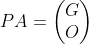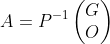，证毕！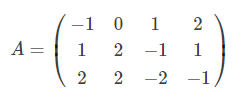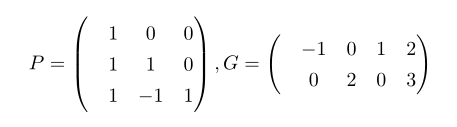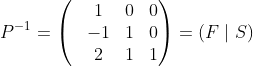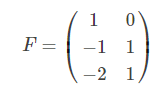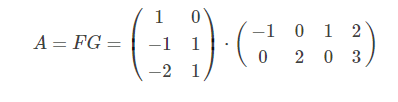1.2  QR分解(UR分解)

设矩阵A∈Rn*n满秩矩阵,则A可以唯一的分解为A=QR。QR分解本质是Gram–Schmidt正交化，当矩阵为复数时，有分解A=UR,此时称为UR分解R是一个正线上三角矩阵：R对角线对角线以上数据都是正数；Q是一个标准正交矩阵，当矩阵为复数时，U是酉矩阵。标准正交矩阵是酉矩阵的一种，酉矩阵是一个对称矩阵，有以下性质：

U=UT

UUT=I。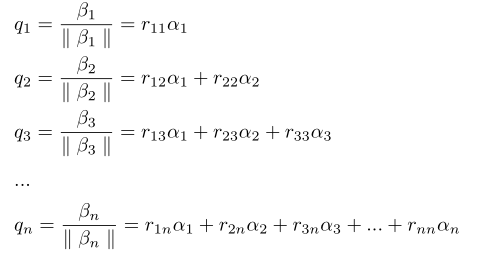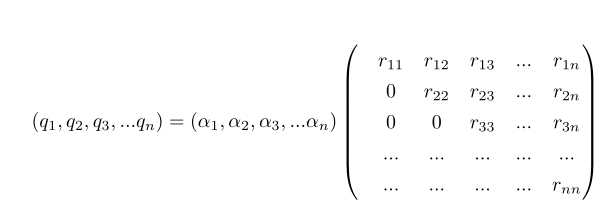以上讨论矩阵A是一个满秩的方阵，当矩阵A秩为r的列满秩矩阵时，即A∈Rm*r,rank(A)=r时，有A=QR,其中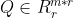，R为正线上三角矩阵;而当A是行满秩矩阵时，即A∈Rr*n,rank(A)=r时,有A=LQ, 其中,L是一个正线下三角矩阵。如果A是一个常规矩阵，且A∈Rm*n,秩r=rank(A)≦min{m,n}时:

A=Q1R1L2R2

```import numpy as np
import  math
import scipy.linalg
#QR分解
def gram_schmidt(A):
"""Gram-schmidt正交化"""
Q=np.zeros_like(A)
cnt = 0
for a in A.T:
u = np.copy(a)
for i in range(0, cnt):
u -= np.dot(np.dot(Q[:, i].T, a), Q[:, i]) # 减去待求向量在以求向量上的投影
e = u / np.linalg.norm(u)  # 归一化
Q[:, cnt] = e
cnt += 1
R = np.dot(Q.T, A)
return  Q, R
if __name__=='__main__':
A = np.array([[6, 5, 9], [5, -1, 6], [5, 1, 4], [0, 4,-4]], dtype=float)
Q, R=gram_schmidt(A)
print(A)
print('='*100)
print(Q)
print('=' * 100)
print(R)```

矩阵A是一般矩阵，A∈Rm*n,秩为rank(A)=r，r≦min{m,n},由线性代数知，ATA是一个n阶方阵，且秩也是r。再引入n个标准正交基向量：v1,v2,v3,....vn，其中v1,v2,v3,....vr是矩阵ATA的特征向量，下面线性映射把由v1,v2,v3,....vn这n个基表示的n维空间映射为另一个n维线性空间：

 上一篇  SVM支持向量机详解 下一篇 逻辑回归详解 评论区